### 3.1 Content-Based Retrieval of Multimedia Information

The content-based information retrieval (CBIR) of multimedia information, e.g., images, sounds, videos, etc., is one of the most important components in large-scale video archive systems. In order to achieve the content-based information retrieval, it is necessary to understand the contents of multimedia information. The most typical case is to express the contents by some feature vectors. In this case, the feature is an approximation of contents and information is retrieved based on the similarity of features assuming that the contents having similar features should be similar to each other. The dissimilarity between two feature vectors is often measured by the Euclidean distance. Once a query vector is specified, such a vector that is the most similar to the query vector is found by the nearest-neighbor search. With this approach, various kinds of systems have been developed. For example, QBIC  is well known as a typical example of the content-based image retrieval system. The practical effectiveness of this approach has been demonstrated by such prototype systems. However, this approach has two difficulties. Firstly, the feature vector is just an approximation of contents. Therefore, the content is retrieved based on the nearest-neighbor search of feature vectors. Secondly, the dimensionality of feature vectors tends to be quite high (e.g., 64 and 256 dimensional vectors are used by QBIC for representing the hue of colors). Thus, fast nearest-neighbor search of high-dimensional vectors is essential to CBIR. In order to alleviate these difficulties, multidimensional indexing methods have been studied by database system researchers. These methods are supposed to take an important role in building large-scale video archive systems which need to process a huge amount of data in a practical processing time.

In this section, we demonstrate the efficiency of multidimensional indexing methods. First, we describe an example of such indexing methods, the SR-tree , which we designed for the efficient similarity retrieval of high dimensional feature vectors. Then, we demonstrate the efficiency of the SR-tree with applying it to face sequence matching.

### 3.2 Multidimensional Index Structures

###### R-Tree and Its Variants

In the database system literature, quite a few index structures have been proposed for high-dimensional indexing. The R-tree  and its variant R*-tree , which were originally designed as a spatial index structure for geography and CAD databases, are used as an index structure for the feature space of the similarity image retrieval . More recently, some index structures, e.g., the SS-tree  and the SR-tree , etc., have been proposed especially for the acceleration of the nearest-neighbor search .

These index structures divide the search space into a hierarchy of regions (Figure 27.5). In addition to the R-tree, the k-d tree and the quadtree are also well-known hierarchical data structures. These structures differ in the way of dividing the search space. The k-d tree and the quadtree divide the search space into disjoint regions. The k-d tree locates the split plane adaptively based on the data distribution, while the quadtree locates the split plane regularly. On the other hand, the R-tree divides the search space by minimum bounding rectangles with allowing the overlap between them. Among these structures, the R-tree is the most efficient for dynamic updates. With allowing overlap between minimum bounding rectangles, the R-tree is kept balanced even after dynamic updates. Therefore, the R-tree is regarded as one of the most suitable index structures for the online database applications.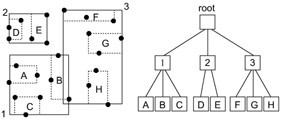Figure 27.5: Structure of the R-tree.

###### SR-Tree: An Index Structure for Nearest Neighbor Queries

The way of the region split has great influence on the performance of index structures. Both the SS-tree and the SR-tree are derived from the R-tree; thus they have the similar structure with the R-tree. However, the SS-tree and the SR-tree have better performance than the R-tree with respect to the nearest-neighbor search. This performance improvement comes from the modification of the region split algorithm. The tree construction algorithm is common to the SS-tree and the SR-tree. It is originally designed for the SS-tree; the SR-tree borrows it from the SS-tree. This algorithm splits regions based on the variance of coordinates. When a node is to be split, the coordinate variance on each dimension is calculated and the dimension with the largest variance is chosen for the split dimension. According to the performance evaluation, it is shown that this algorithm generates regions with shorter diameters than the algorithm of the R*-tree does .

The difference between the SS-tree and the SR-tree is entries of internal nodes. An internal node of the SS-tree has the following entries: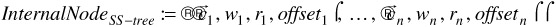where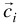(1in) denotes the centroid of the i-th child node, i.e., the centroid of the points contained in the subtree whose root node is the i-th child node, wi (1 in) denotes the weight of the i-th child node, i.e., the number of the points in the subtree, and ri (1 in) denotes the radius of the minimum bounding sphere that covers all points in the subtree and whose center is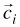. Thus, the SS-tree employs minimum bounding spheres instead of minimum bounding rectangles. The tree structure of the SS-tree corresponds to the hierarchy of spherical regions (Figure 27.6). The centroidsand the weights wl,...,wn play an important role in the variance-based region split algorithm. They make it possible to estimate the variance of the data distribution at each internal node. The SS-tree outperforms the R-tree by virtue of the variance-based algorithm.Figure 27.6: Structure of the SS-tree.

The SR-tree is an enhancement of the SS-tree. It employs both the bounding sphere and the bounding rectangle as follows: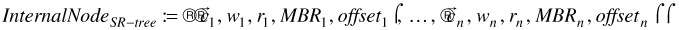where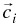, wi , and ri (1 i n) are the same with those of the SS-tree, and MBRi (1in) denotes the minimum bounding rectangle of the i-th child node. Thus, the difference between the SS-tree and the SR-tree is the addition of MBRi. Each sub-region of the SR-tree is determined by the intersection of the bounding sphere (given by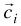and ri) and the bounding rectangle (given by MBRi). The tree structure of the SR-tree corresponds to the hierarchy of such regions (Figure 27.7). The experimental evaluation has proven that the incorporation of minimum bounding rectangles improves the performance of nearest-neighbor search because minimum bounding rectangles reduce the region size of each node .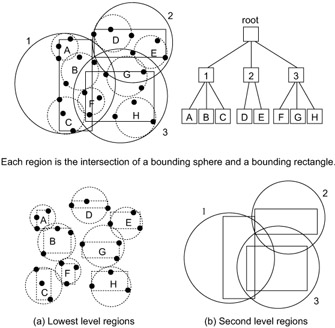Figure 27.7: Structure of the SR-tree.

###### Nearest-Neighbor Search with Multidimensional Index Structures

The multidimensional index structures can accelerate the nearest-neighbor search since the search can be accomplished with investigating a limited number of nodes (i.e., regions). This could reduce the search space significantly and would be much faster than investigating every feature vector one by one. The search starts from the root node and visits regions in ascending order of the distance from the query point. On visiting each region, the candidate of the nearest neighbor is determined by choosing the nearest point encountered so far. The search continues as long as there remains such a region that is not visited so far and that is closer than the candidate. The search terminates when no region remains to be visited. The final candidate is the answer of the query. The number of nodes to be visited is dependent on various circumstances, e.g., distribution of the data set, location of the query, and the characteristics of the index structure, etc. In the ideal case, the nearest neighbor can be found with traversing the tree from the root node to a leaf straightly. In this case, the search cost is logarithmic to the size of the database (i.e., the number of feature vectors). The results on the performance evaluation of the SR-tree can be found in .

### 3.3 Efficient Implementation of Face Sequence Matching

###### Similarity Retrieval with Colored Nearest-Neighbor Search

In the case of the similarity retrieval of images, one feature vector is extracted from each image and nearest-neighbor search is conducted with comparing one vector to another. On the other hand, some multimedia information, e.g., videos, permits us to extract multiple feature vectors from each piece of multimedia information. In this case, the colored nearest-neighbor search can be employed for improving the accuracy of the similarity retrieval.

For example, in Figure 27.8, multiple feature vectors which are obtained from each sequence of video frames compose a colored set of feature vectors. The similarity of two vector sets can be measured by the distance of their closest pair as shown in Figure 27.8. In the case of the similarity retrieval of video sequences, the closest pair of two feature vector sets corresponds to the pair of the most similar video frames that are shared by two video sequences. This type of the similarity measurement has been used by the face sequence matching described below and the appearance matching by Nayar et al. .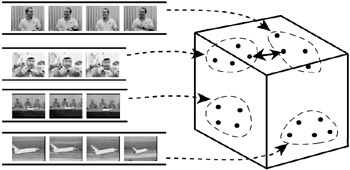Figure 27.8: Similarity retrieval of video frame sequences based on the closest pair.

However, this similarity measurement is rather computation intensive. The naive method of finding the closest pair of two vector sets is to compare every combination of vectors. In this case, the computational cost is a serious problem.

Suppose that we have a database containing N video sequences and retrieve such a sequence that is the most similar to a given query. If one vector is extracted from each video sequence, the most similar one can be found by N comparisons of feature vectors. On the other hand, if M feature vectors are extracted from each sequence, M M N comparisons are required to find the closest pair having the shortest distance. Of course, this estimation based on the naive implementation is like the linear scan but it is obvious that the computational cost is a serious problem for the colored nearest-neighbor search. Therefore, it is necessary to reduce the search cost in order to apply the colored nearest-neighbor search to large-scale video databases. From this perspective, we applied the SR-tree to the colored nearest-neighbor search.

###### Colored NN Search with Multidimensional Index Structures

The colored nearest-neighbor search can be easily conducted with multidimensional index structures by the extension of the non-colored nearest-neighbor search described above. The fundamental flow is similar to the non-colored nearest-neighbor search: nodes of a tree are sorted in ascending order of the distance to the given query and the closest node is visited before the others. For the colored nearest-neighbor search, a query is given by the set of vectors. Therefore, when computing the distance from the query to a node entry (i.e., a child node or a vector), we must choose such a query vector that is closest to the entry and then compute the distance from the vector to the entry. The search consists of two steps as the non-colored nearest-neighbor search. Firstly, the leaf node that is the closest to the query vectors is visited to obtain the initial candidate of the nearest neighbor. Secondly, the search continues with visiting such a node that is closer to the query than the candidate. Every time a node is visited, the candidate is updated. The search terminates when there is no node that is closer to the query than the candidate is. The final candidate is the answer of the query.

In the case of finding k nearest neighbors, k candidates are kept during the search operation in the same way as the non-colored nearest-neighbor search. However, in the colored nearest-neighbor search, at most one vector should be selected for a candidate from each vector set. Therefore, when a new candidate is found, we must test whether the current candidate set contains such a vector that belongs to the same set with the one of the new candidate. If not, we can add the new candidate to the candidate set; if it does, we need to compare the new candidate with the vector belonging to the same set and then only the closer vector should be included in the candidate set.

###### Performance Evaluation with Face Sequence Matching

In order to evaluate the effectiveness of the multidimensional index structures, we conducted an experiment of face sequence matching. A face sequence consists of the face images extracted from videos. Even if two face sequences are of the same person, they involve the variation in diverse aspects, e.g., facial expression, lighting conditions, etc. Therefore, the closest pair of two face sequences corresponds to the pair of the most similar faces that are shared by those sequences.

The experiment was conducted with CNN Headline News video. Six data sets are composed by sampling 100, 200, 300, 400, 500, and 566 sequences at random from 566 sequences extracted from the video. The numbers of face images contained in the data sets are 1414, 2599, 4174, 5504, 7257, and 8134 respectively. The SR-tree is used as a multidimensional index structure.

We measured the CPU time and the disk access (the amount of disk read) of finding such a sequence that is closest to a given query sequence. Every sequence in a data set is chosen for a query and the average of them is taken as the experiment result. Programs are implemented in C++. Tests are conducted on a Sun Microsystems workstation, Ultra 60 (CPU: UltraSPARC-II 360MHz, main memory: 512Mbytes, OS: Solaris 2.6). Figure 27.9 shows the measured performance. For comparison, the result of the implementation with the linear scan is also plotted. The horizontal axis indicates the number of face images in a data set. This number corresponds to the size of a database. The vertical axis on the left indicates the CPU time and the one on the right the disk access. The effectiveness of the SR-tree is more significant in the disk access. When the number of face images is 8134, the disk access of the SR-tree is only 18 % of that of the linear scan. This demonstrates that the SR-tree successfully reduces the search space with splitting the data space into the hierarchy of regions. This clearly shows the advantage of multidimensional index structures. The CPU time of the SR-tree is also much smaller than that of the linear scan; the former is 29 % of the latter. In addition, the ratio of the SR-tree to the linear scan, i.e., "SR-tree / Linear Scan" in the figure, decreases as the database size increases. This demonstrates the scalability of the proposed method and implies its effectiveness for large-scale databases.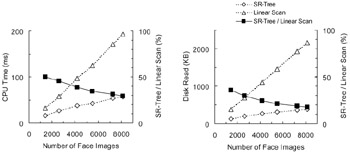Figure 27.9: Performance of the SR-tree with face sequence matching.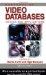Handbook of Video Databases: Design and Applications (Internet and Communications)
ISBN: 084937006X
EAN: 2147483647
Year: 2003
Pages: 393